# Homology groups and fundamental group need not determine homotopy groups

Jump to: navigation, search

## Statement

It is possible to have two path-connected spaces$M_1$ and$M_2$ such that:

•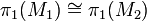$\pi_1(M_1) \cong \pi_1(M_2)$, i.e.,$M_1$ and$M_2$ have isomorphic Fundamental group (?)s.
•$H_k(M_1) \cong H_k(M_2)$ for each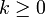$k \ge 0$, i.e.,$M_1$ and$M_2$ have isomorphic Homology group (?)s in each homology.
• There exists some$k \ge 2$ such that the Homotopy group (?)s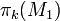$\pi_k(M_1)$ and$\pi_k(M_2)$ are not isomorphic.

In fact, we can choose both$M_1$ and$M_2$ to be Simply connected space (?)s, i.e., they both have trivial fundamental group.

## Facts used

1. Fundamental group of wedge sum relative to basepoints with neighborhoods that deformation retract to them is free product of fundamental groups

## Proof

Further information: wedge sum of 2-sphere and 4-sphere, complex projective plane

Let$M_1 = S^2 \vee S^4$ be the wedge sum of the 2-sphere$S^2$ and the 4-sphere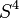$S^4$ and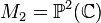$M_2 = \mathbb{P}^2(\mathbb{C})$. They both have the same homology groups: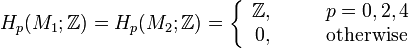$H_p(M_1;\mathbb{Z}) = H_p(M_2;\mathbb{Z}) = \left\lbrace\begin{array}{rl} \mathbb{Z}, & \qquad p = 0,2,4 \\ 0, & \qquad \text{otherwise}\\\end{array}\right.$

Also, they are both simply connected spaces ($M_1$ is simply connected by Fact (1), for$M_2$, see homology of complex projective space).

However, they do not have the same isomorphism class of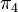$\pi_4$: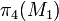$\pi_4(M_1)$ is nontrivial whereas$\pi_4(M_2)$ is trivial. For this, note that:

• There is a retraction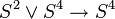$S^2 \vee S^4 \to S^4$ that sends all points in the$S^4$ piece to themselves and all points in the$S^2$ piece to the point of wedging. This retraction induces a retraction on each homotopy group, so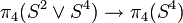$\pi_4(S^2 \vee S^4) \to \pi_4(S^4)$ is a retraction. In particular, since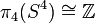$\pi_4(S^4) \cong \mathbb{Z}$ is nontrivial,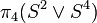$\pi_4(S^2 \vee S^4)$ is also nontrivial.
•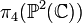$\pi_4(\mathbb{P}^2(\mathbb{C}))$ is the trivial group, based on the homotopy of complex projective space.